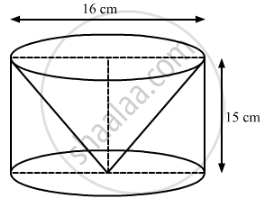Advertisement Remove all ads

# From a Solid Cylinder Whose Height is 15 Cm and Diameter 16 Cm, a Conical Cavity of the Same Height and Same Diameter is Hollowed Out. Find the Total Surface Area of the Remaining Solid. - Mathematics

Sum

From a solid cylinder whose height is 15 cm and diameter 16 cm, a conical cavity of the same height and same diameter is hollowed out. Find the total surface area of the remaining solid. (Use π = 3.14)

Advertisement Remove all ads

#### SolutionWe have,

Height of the cylinder = Height of the cone = h = 15 cm and

Radius of thecylinder = Radius of the cone = r = 16/2 = 8  "cm"

Also, the slant height of the cone, l =sqrt("h"^2 + "r"^2)

=sqrt(15^2 + 8^2)

=sqrt(225+64)

=sqrt(289)

= 17 cm,

Now,

Then total surface area of the remaining solid = CSA of the cone + CSA of the cone + Area of the base

=πrl+ 2πrh + πr

= πr (l + 2h + r)

= 3.14 × 8× (17 + 2 × 15 + 8)

= 3.1× 8 × 55

= 1381.6 cm2

So, the total surface area of the remaining solid is 1381.6 cm2.

Disclaimer: The answer given in the textbook is incorrect. The same has been corrected above.

Is there an error in this question or solution?
Advertisement Remove all ads

#### APPEARS IN

RS Aggarwal Secondary School Class 10 Maths
Chapter 19 Volume and Surface Area of Solids
Formative Assessment | Q 17 | Page 938
Advertisement Remove all ads
Advertisement Remove all ads
Share
Notifications

View all notifications

Forgot password?